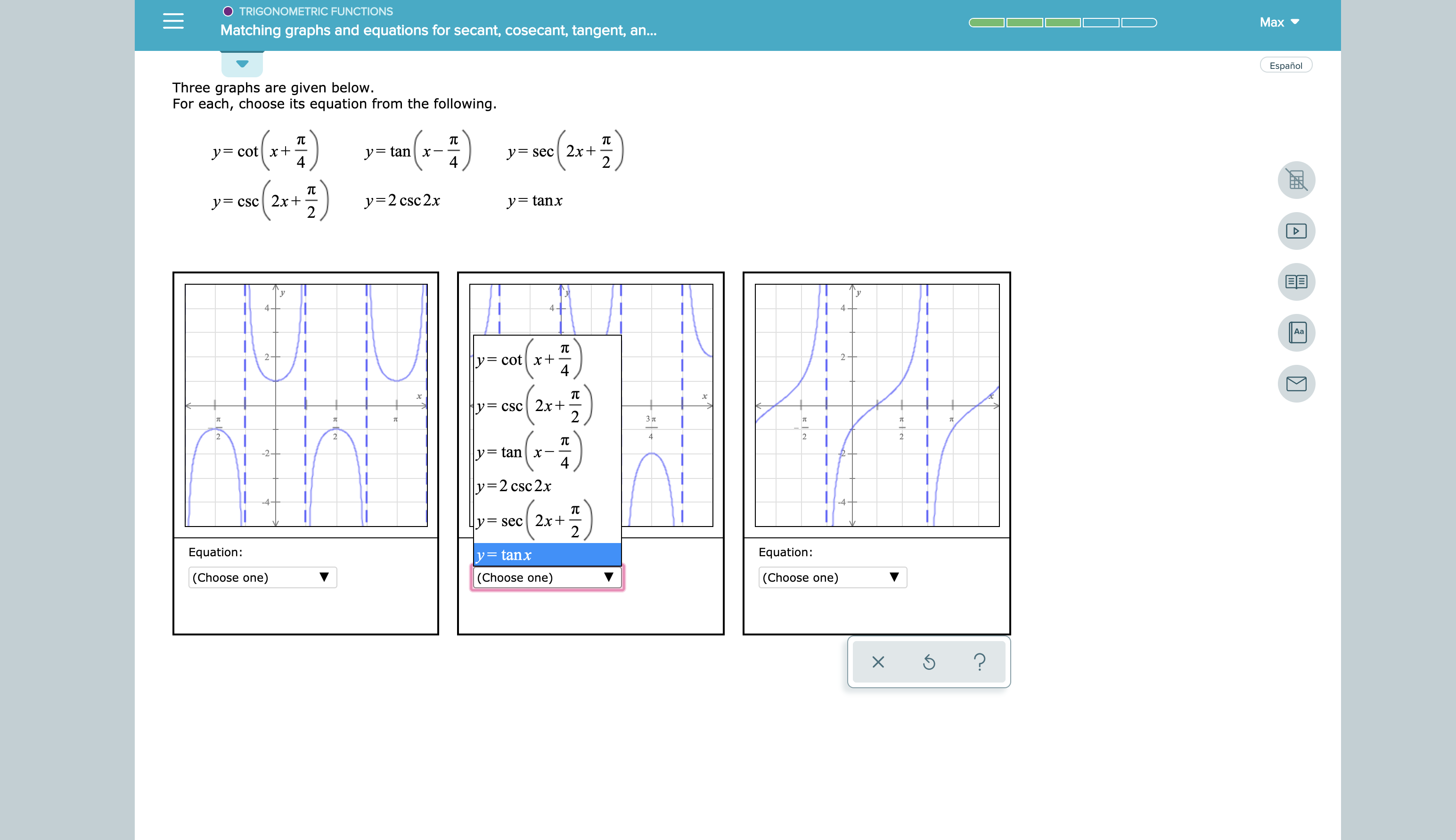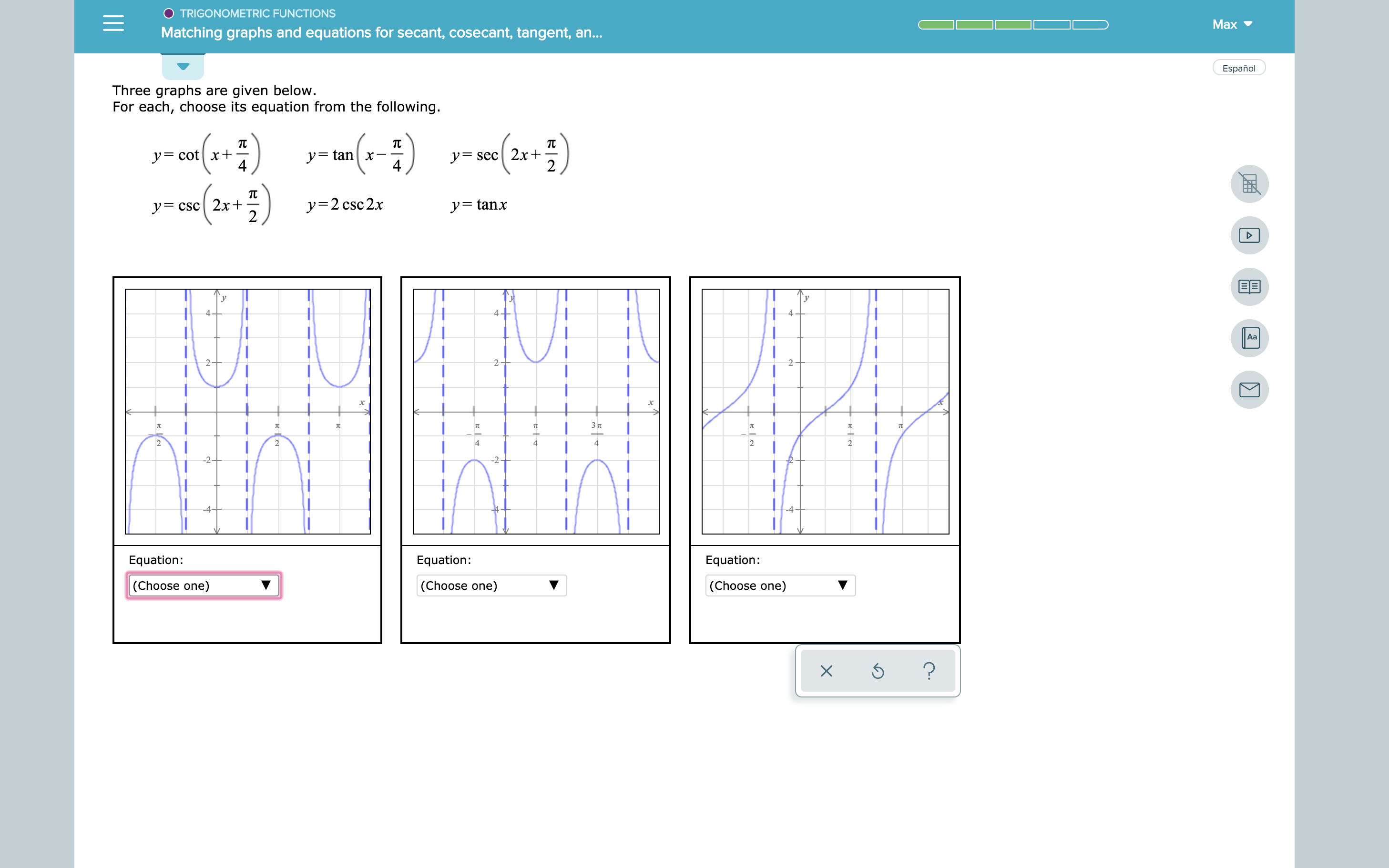# TRIGONOMETRIC FUNCTIONS Max Matching graphs and equations for secant, cosecant, tangent, a... Español Three graphs are given below. For each, choose its equation from the following. т y=cotx+ 4 y=tan x 4 у- sec| 2x+ yCSc 2x+ 2 y=2 csc2x y= tanx 4- 4 4 Aa y= cotx+ 4 2 y= csc 2x+ 2 3л 2 2 4 2 2 TC y= tanx 4 -2+ y=2 csc2x -4- -4 т = sec 2x+ 2 Equation: Equation: y=tanx (Choose one) (Choose one) (Choose one) ? O TRIGONOMETRIC FUNCTIONS Mаx Matching graphs and equations for secant, cosecant, tangent, an... Español Three graphs are given below. For each, choose its equation from the following. т y=sec2x+ 2 ycotx+ 4 y= tanx 4 yCSc 2x+ 2 y=2 csc2x y=tanx 4+ 4 Аа 2- 2 2 3л TU TC 2 2 4 4 4 2 2 2 -2 Equation: Equation: Equation: (Choose one) (Choose one) (Choose one) ?

Question

See attachmenthelp_outlineImage TranscriptioncloseTRIGONOMETRIC FUNCTIONS Max Matching graphs and equations for secant, cosecant, tangent, a... Español Three graphs are given below. For each, choose its equation from the following. т y=cotx+ 4 y=tan x 4 у- sec| 2x+ yCSc 2x+ 2 y=2 csc2x y= tanx 4- 4 4 Aa y= cotx+ 4 2 y= csc 2x+ 2 3л 2 2 4 2 2 TC y= tanx 4 -2+ y=2 csc2x -4- -4 т = sec 2x+ 2 Equation: Equation: y=tanx (Choose one) (Choose one) (Choose one) ? fullscreenhelp_outlineImage TranscriptioncloseO TRIGONOMETRIC FUNCTIONS Mаx Matching graphs and equations for secant, cosecant, tangent, an... Español Three graphs are given below. For each, choose its equation from the following. т y=sec2x+ 2 ycotx+ 4 y= tanx 4 yCSc 2x+ 2 y=2 csc2x y=tanx 4+ 4 Аа 2- 2 2 3л TU TC 2 2 4 4 4 2 2 2 -2 Equation: Equation: Equation: (Choose one) (Choose one) (Choose one) ? fullscreen

### Want to see this answer and more?

Experts are waiting 24/7 to provide step-by-step solutions in as fast as 30 minutes!*

*Response times vary by subject and question complexity. Median response time is 34 minutes and may be longer for new subjects.
Tagged in
MathCalculus

### Other